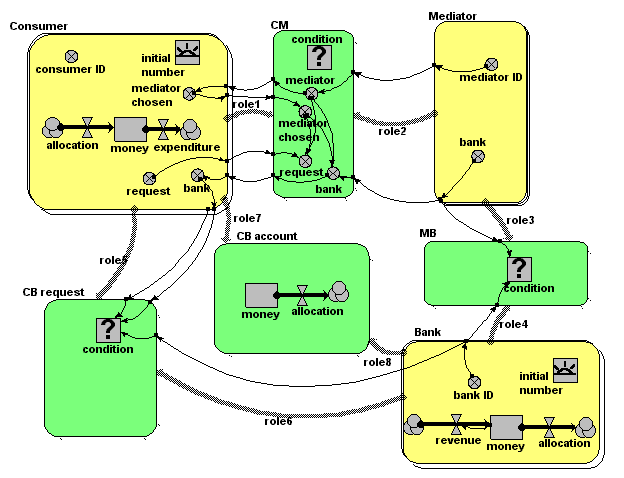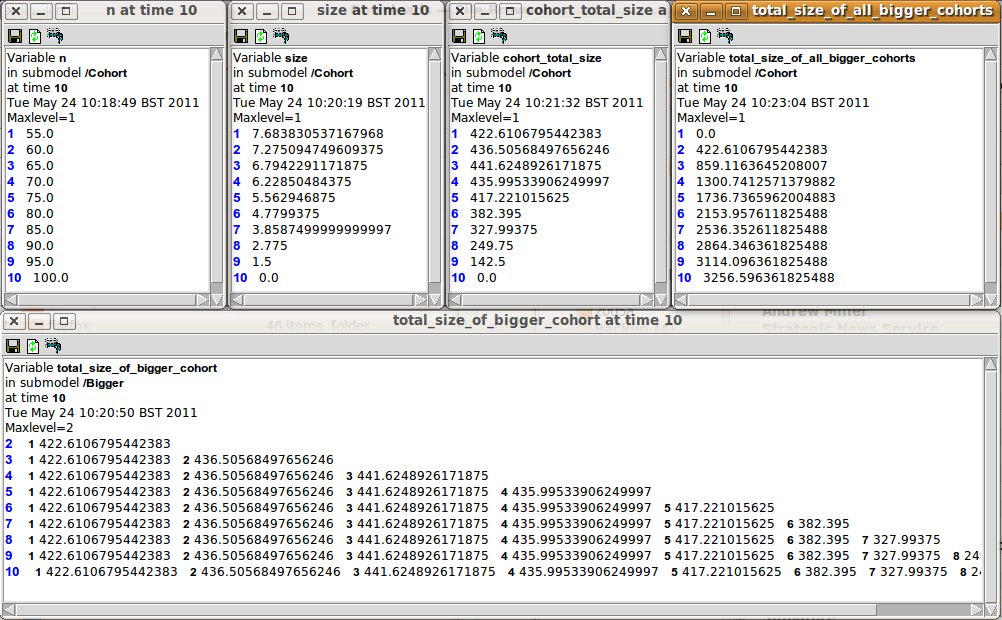# Population submodel

## Assigning rank order to the instances of a population submode

ModelId:
ranking1
SimileVersion:
3.1

There are a number of situations where you might want to rank the members (instances) of a population submodel in order, according to some criterion.

Category:
Technique
Equations:

Equations in submodel 'individual'

attribute = rand_const(0,100)
rank = count({one_role2})+1
where: {one_role1} = ../ranking/one (to individual in role1)
creation: initial number = 10

Equations in submodel 'ranking'
condition = (attribute_role1>attribute_role2)
where:
attribute_role1 = ../individual/attribute (from individual in role1)
attribute_role2 = ../individual/attribute (from individual in role2)]
one = 1

Model tags:

## Banker/broker/consumer multi-agent model

ModelId:
dave1
SimileVersion:
3.1+

This model was developed in relation to the SLIE project (Sustainable Lifecycles for Information Ecosystems). That project is based on agent-based approaches: this model aims to explore the extent to which such models can be implemented in the Simile environment.Equations:

Equations in submodel Bank

creation: initial number = 2
compartment: money = 100000
flow: revenue = 0.1*money
flow: allocation = 0
variable: bank ID = index(1)

Equations in submodel Consumer
creation: initial number = 4
compartment: money = 0
flow: allocation = 0
flow: expenditure = 100
variable: consumer ID = index(1)
variable: request = (if rand_var(0,1)<0.2 then 1 else 0)
variable: mediator chosen = greatest({mediator})
variable: bank = sum({bank})

Equations in submodel Mediator
variable: mediator ID = index(1)
variable: bank = -1*floor(rand(1,2.9999))

Equations in submodel CM
condition: condition = (rand_const(0,1)<0.5)
variable: request = (if mediator==mediator_chosen then request else 0)
variable: mediator = mediator_id
variable: mediator chosen = mediator_chosen [in submodel Consumer]
variable: bank = (if mediator==mediator_chosen then bank else 0)

Equations in submodel MB
condition: condition = (bank==bank_id)

Equations in submodel CB request
condition: condition = (bank==bank_id&&request>0)

Equations in submodel CB account
compartment: money = 0

## Skeleton cohort model

ModelId:
cohort1
SimileVersion:
5.97

This example shows how to implement a basic cohort model in Simile.

A cohort model is one way of modelling a population. The individuals are grouped into cohorts, typically on the basis that they were born at the same time. Each cohort has as a minimum a variable representing the number of individuals in that cohort, along with possibly other cohort-specific attributes, such as the average size of the individuals in the cohort.

Equations:

Variable: n_total = sum({n})

Where: {n} = Cohort/n

Variable: population_total_size = sum({cohort_total_size})
Where: {cohort_total_size} = Cohort/cohort_total_size

--------------------------------------------------------------
Submodel Bigger

Submodel "Bigger" is an association submodel between "Cohort" and itself with roles "other" and "me".

Condition: condition = other_size>me_size
Where:
me_size = Value(s) of ../Cohort/size from submodel "Cohort" in role "me"
other_size = Value(s) of ../Cohort/size from submodel "Cohort" in role "other"

Variable: total_size_of_bigger_cohort = other_cohort_total_size
Where:
me_cohort_total_size = Value(s) of ../Cohort/cohort_total_size from submodel "Cohort" in role "me"
other_cohort_total_size = Value(s) of ../Cohort/cohort_total_size from submodel "Cohort" in role "other"

-------------------------------------------------------------------------
Submodel Cohort

Submodel "Cohort" is a population submodel.

Compartment: n
Initial value = 100
Rate of change = - loss

Compartment: size
Initial value = 0
Rate of change = + growth

Flow: growth = gr*(1-size/max_size)

Flow: loss = 5

Immigration: create_new_cohort = 1

Loss: remove_cohort = n<=0

Variable: cohort_total_size = n*size

Variable: gr = 1.5

Variable: max_size = 10

Variable: total_size_of_all_bigger_cohorts = sum({cohort_total_size_of_bigger_me})
Where:
{cohort_total_size_of_bigger_me} = ../Bigger/total_size_of_bigger_cohort for submodel "Cohort" in role "me"

Results:

The results shown here illustrate the basic functioning of this model.

The following figure shows a set of panels, each one showing the values for a specified variable at time 10. These were made using Simile's 'snapshot' tool, and enable us to trace the values as they are processed in the model.The first 3 panels on the top row show, for each of the 10 cohorts created up to this time, the number of tress (n) and the size of the average tree (size) in the cohort, along with the total size (n x size). You can see that the number of tress declines in a cohort declines by 5 each time step, and that the average tree in a cohort grows at a reducing rate as the cohort gets older.

The bottom window shows the total_size values for the individual cohorts that are bigger than the specified cohort. Thus, cohort 2 only has one value (since there is only one bigger than it is), while cohort 3 has 2 values, and so on.

The top-right window shows 'total_size_of_all_bigger_cohorts' - the sum (along a row) for al the total_size values of bigger cohorts for a particular cohort. This is the key value which enables the impact of bigger cohorts on the growth of a cohort to be modelled.

The graph below shows how the 'total_size_of_all_bigger_cohorts' changes over time, for each cohort. The lowest curve is for the second cohort (since the first cohort has nothing bigger than it. Each successive cohort starts off at a higher value (since the total size of cohorts above it is increasing), until we get to the point where each newly-created cohort starts off with the same total_size above it, which decreases as that cohort in turn increases in size.